Quick Search for:  in language:
Code/Articles  |  Newest/Best  |  Community  |  Jobs  |  Other  |  Goto  |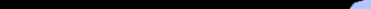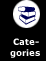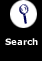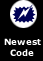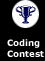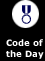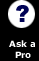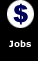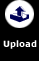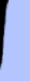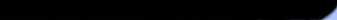Perl Stats

Code: 74,273. lines
Jobs: 25. postings

How to support the site

 You are in:***Site Home***.NetASP / VbScriptC / C++Cold FusionDelphiJava / JavascriptLISPPerlPHPSQLVisual Basic

Latest Code Ticker for Perl.
 Simple Perl PingBy John Hass on 12/29Very basic login script template with cookiesBy Aaron L. Anderson on 12/29

Daily Code Email

 Affiliate Sites

QB Passing Calculator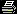Print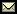Email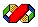Submitted on: 9/18/2003 11:59:55 PM By: Randy McCleary  Level: IntermediateUser Rating: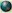By 3 Users Compatibility:Active Perl specificUsers have accessed this code 1319 times. (About the author)

This is a Football Quarterback Passing Calculator.This application will calculate completion percentage, yards per Attempt, yards per completion, and passing Efficiency

code:
Can't Copy and Paste this?
 Terms of Agreement:    By using this code, you agree to the following terms...    1) You may use this code in your own programs (and may compile it into a program and distribute it in compiled format for languages that allow it) freely and with no charge.    2) You MAY NOT redistribute this code (for example to a web site) without written permission from the original author. Failure to do so is a violation of copyright laws.    3) You may link to this code from another website, but ONLY if it is not wrapped in a frame.  4) You will abide by any additional copyright restrictions which the author may have placed in the code or code's description. ``` =************************************** = Name: QB Passing Calculator = Description:This is a Football Quarter = back Passing Calculator.This application = will calculate completion percentage, ya = rds per Attempt, yards per completion, a = nd passing Efficiency = By: Randy McCleary = =This code is copyrighted and has = limited warranties.Please see http://w = ww.Planet-Source-Code.com/vb/scripts/Sho = wCode.asp?txtCodeId=541&lngWId;=6 =for details. =************************************** #!/usr/bin/perl -w ###################################################################### ## This is a Football Quarterback Passing Calculator. ## This application will calculate completion percentage, yards ##per Attempt, yards per completion, and passing Efficiency ###################################################################### use strict; use Tk; use Tk::Dialog; use Number::Format qw(:subs); require Tk::LabFrame; my \$frmMain = MainWindow->new; \$frmMain->title("QB Passing Calculator"); \$frmMain->geometry("461x248+8+8"); my \$fraInput = \$frmMain->LabFrame( -label => "Input QB Passing Stats", -labelside => "acrosstop"); my \$fraDisplay = \$frmMain->LabFrame( -label => "Calculated Stats", -labelside => "acrosstop"); ###################################################################### ## Create the labels and Text Entries to put into the frame fraInput ###################################################################### ############################################## ## Here is where we create the Labels and ##set the attributes to the labels that ##Request the user to input the values use ##to calculate the stats. ############################################## my \$lblAttempts = \$fraInput->Label( -anchor => "w", -borderwidth => 1, -text => "Attempts:", -background => "#D4D0C8", -cursor => "", -font => "Tahoma 9 bold", -foreground => "#0000A0", -relief => "flat" )->pack; my \$lblCompletions = \$fraInput->Label( -anchor => "w", -borderwidth => 1, -text => "Completions:", -background => "#D4D0C8", -cursor => "", -font => "Tahoma 9 bold", -foreground => "#0000A0", -relief => "flat" )->pack; my \$lblYards = \$fraInput->Label( -anchor => "w", -borderwidth => 1, -text => "Total Yards:", -background => "#D4D0C8", -cursor => "", -font => "Tahoma 9 bold", -foreground => "#0000A0", -relief => "flat" )->pack; my \$lblTDs = \$fraInput->Label( -anchor => "w", -borderwidth => 1, -text => "Pass TD's:", -background => "#D4D0C8", -cursor => "", -font => "Tahoma 9 bold", -foreground => "#0000A0", -relief => "flat" )->pack; my \$lblInts = \$fraInput->Label( -anchor => "w", -borderwidth => 1, -text => "Interceptions:", -background => "#D4D0C8", -cursor => "", -font => "Tahoma 9 bold", -foreground => "#0000A0", -relief => "flat" )->pack; ############################################## ## Here is where we create the Text Entries ##and set the attributes to the Entries ##that will grab the input values to do ##the calculations. ############################################## my \$txtAttempts = \$fraInput->Entry( -borderwidth => 1, -cursor => "", -font => "Tahoma 8 normal", -relief => "sunken" )->pack; my \$txtCompletions = \$fraInput->Entry( -borderwidth => 1, -cursor => "", -font => "Tahoma 8 normal", -relief => "sunken" )->pack; my \$txtYards = \$fraInput->Entry( -borderwidth => 1, -cursor => "", -font => "Tahoma 8 normal", -relief => "sunken" )->pack; my \$txtTDs = \$fraInput->Entry( -borderwidth => 1, -cursor => "", -font => "Tahoma 8 normal", -relief => "sunken" )->pack; my \$txtInts = \$fraInput->Entry( -borderwidth => 1, -cursor => "", -font => "Tahoma 8 normal", -relief => "sunken" )->pack; ############################################## ## Here is where we set the placement of ##all the labels that request the input ##data to be entered. ############################################## \$lblAttempts->place( -width => 96, -height => 24, -x => 8, -y => 8); \$lblCompletions->place( -width => 96, -height => 24, -x => 8, -y => 37); \$lblYards->place( -width => 96, -height => 24, -x => 8, -y => 66); \$lblTDs->place(-width => 96, -height => 24, -x => 8, -y => 95); \$lblInts->place( -width => 96, -height => 24, -x => 8, -y => 124); ############################################## ## Here is where we set the placement of ##all the Text Entries that are used to ##accept the Input Data to process. ############################################## \$txtAttempts->place( -width => 64, -height => 24, -x => 110, -y => 8); \$txtCompletions->place( -width => 64, -height => 24, -x => 110, -y => 37); \$txtYards->place( -width => 64, -height => 24, -x => 110, -y => 66); \$txtTDs->place( -width => 64, -height => 24, -x => 110, -y => 95); \$txtInts->place( -width => 64, -height => 24, -x => 110, -y => 124); ###################################################################### ## End of the creation of fraInput ###################################################################### ###################################################################### ## Create the labels and place them into the frame fraDisplay ###################################################################### ############################################## ## Here is where we create the Labels and ##set the attributes to the labels that ##Displays the names of the Stats. ############################################## my \$lblCompPct = \$fraDisplay->Label( -anchor => "w", -borderwidth => 1, -text => "Completion %:", -background => "#D4D0C8", -cursor => "", -font => "Tahoma 9 bold", -foreground => "#0000A0", -relief => "flat" )->pack; my \$lblYrdAttempt = \$fraDisplay->Label( -anchor => "w", -borderwidth => 1, -text => "Yards per Attempt:", -background => "#D4D0C8", -cursor => "", -font => "Tahoma 9 bold", -foreground => "#0000A0", -relief => "flat" )->pack; my \$lblYrdComp = \$fraDisplay->Label( -anchor => "w", -borderwidth => 1, -text => "Yards / Completion:", -background => "#D4D0C8", -cursor => "", -font => "Tahoma 9 bold", -foreground => "#0000A0", -relief => "flat" )->pack; my \$lblPassEff = \$fraDisplay->Label( -anchor => "w", -borderwidth => 1, -text => "Passing Efficiency:", -background => "#D4D0C8", -cursor => "", -font => "Tahoma 9 bold", -foreground => "#0000A0", -relief => "flat" )->pack; ############################################## ## Here is where we create the Labels and ##set the attributes to the labels that ##will end up displaying the Stats. ############################################## my \$txtCompPct = \$fraDisplay->Label( -anchor => "e", -borderwidth => 1, -text => "", -background => "#FFFFFF", -cursor => "", -font => "Tahoma 8 normal", -highlightbackground => "#FFFFFF", -relief => "solid" )->pack; my \$txtYrdAttempt = \$fraDisplay->Label( -anchor => "e", -borderwidth => 1, -text => "", -background => "#FFFFFF", -cursor => "", -font => "Tahoma 8 normal", -highlightbackground => "#FFFFFF", -relief => "solid" )->pack; my \$txtYrdComp = \$fraDisplay->Label( -anchor => "e", -borderwidth => 1, -text => "", -background => "#FFFFFF", -cursor => "", -font => "Tahoma 8 normal", -highlightbackground => "#FFFFFF", -relief => "solid" )->pack; my \$txtPassEff = \$fraDisplay->Label( -anchor => "e", -borderwidth => 1, -text => "", -background => "#FFFFFF", -cursor => "", -font => "Tahoma 8 normal", -highlightbackground => "#FFFFFF", -relief => "solid" )->pack; ########################################## ## Here is where we set the placement of ##all the labels that displays the ##name of the Stats. ########################################## \$lblCompPct->place( -width => 125, -height => 24, -x => 8, -y => 8); \$lblYrdAttempt->place( -width => 125, -height => 24, -x => 8, -y => 45); \$lblYrdComp->place( -width => 125, -height => 24, -x => 8, -y => 82); \$lblPassEff->place( -width => 125, -height => 24, -x => 8, -y => 119); ########################################## ## Here is where we set the placement of ##all the labels that display the ##Statistics values. ########################################## \$txtCompPct->place( -width => 64, -height => 24, -x => 135, -y => 8); \$txtYrdAttempt->place( -width => 64, -height => 24, -x => 135, -y => 45); \$txtYrdComp->place( -width => 64, -height => 24, -x => 135, -y => 82); \$txtPassEff->place( -width => 64, -height => 24, -x => 135, -y => 119); ###################################################################### ## End of the creation of fraDisplay ###################################################################### \$fraInput->pack; \$fraDisplay->pack; ########################################## ## Here is where we set the placement of ##the two LabelFrames. ########################################## \$fraInput->place( -width => 216, -height => 184, -x => 13, -y => 16); \$fraDisplay->place( -width => 216, -height => 184, -x => 232, -y => 16); ########################################## ## Here is where we create the command ## buttons and set the attributes. ########################################## my \$cmdCalculate = \$frmMain->Button( -activeforeground => "#000000", -background => "#FFFFFF", -borderwidth => 1, -text => "Calculate", -command => sub{Calculate()}, -cursor => "", -font => "Tahoma 10 bold", -foreground => "#820000", -relief => "solid" ); my \$cmdClear = \$frmMain->Button( -activeforeground => "#000000", -background => "#FFFFFF", -borderwidth => 1, -text => "Clear", -command => sub{Clear()}, -cursor => "", -font => "Tahoma 10 bold", -foreground => "#820000", -relief => "solid" ); my \$cmdExit = \$frmMain->Button( -activeforeground => "#000000", -background => "#FFFFFF", -borderwidth => 1, -text => "Exit", -command => [\$frmMain => 'destroy'], -cursor => "", -font => "Tahoma 10 bold", -foreground => "#820000", -relief => "solid" ); ########################################## ## Here is where we set the placement ## of all the command buttons. ########################################## \$cmdCalculate->place( -width => 112, -height => 32, -x => 64, -y => 208); \$cmdClear->place( -width => 96, -height => 32, -x => 184, -y => 208); \$cmdExit->place( -width => 112, -height => 32, -x => 288, -y => 208); MainLoop; ###################################################################### ## Subs Functions below ###################################################################### ################################################################## ## Sub: Calculate ## Description: This sub will get all the input data and does ##the actual calculation and displays the output in the ##Stat Labels. Error Checking is also included. ################################################################## sub Calculate { #################################### ### Define the Calculated variables #################################### my \$dblCompletion = 0; my \$dblYardsPerAtt = 0; my \$dblYardsPerComp = 0; my \$dblPassEff = 0; my \$dialog; #################################### ### Get Input Values #################################### my \$intAttempts = \$txtAttempts->get; my \$intComplete = \$txtCompletions->get; my \$intYards = \$txtYards->get; my \$intTDs = \$txtTDs->get; my \$intInts = \$txtInts->get; #################################### ## Validate the Input Data ## 1. All Data must be numeric ## 2. Passing Attempts and Completions ##must be greater than 0. #################################### if (IsNumeric(\$intAttempts) == 0 || IsNumeric(\$intComplete) == 0) { \$dialog = \$frmMain->Dialog( -title => "QB Passing Calculator: Error", -text => "Invalid Data was entered in Passing Attempts or Completions\nNumber must be numeric.", -buttons => ["OK"]); \$dialog->Show(); } elsif (\$intAttempts == 0 || \$intComplete == 0) { \$dialog = \$frmMain->Dialog( -title => "QB Passing Calculator: Error", -text => "Passing Attempts and Completions must not be equal to 0.", -buttons => ["OK"]); \$dialog->Show(); } elsif (IsNumeric(\$intYards) == 0) { \$dialog = \$frmMain->Dialog( -title => "QB Passing Calculator: Error", -text => "Invalid Data was entered in Total Yards\nNumber must be numeric.", -buttons => ["OK"]); \$dialog->Show(); } elsif (IsNumeric(\$intTDs) == 0) { \$dialog = \$frmMain->Dialog( -title => "QB Passing Calculator: Error", -text => "Invalid Data was entered in Passing TD's\nNumber must be numeric.", -buttons => ["OK"]); \$dialog->Show(); } elsif (IsNumeric(\$intInts) == 0) { \$dialog = \$frmMain->Dialog( -title => "QB Passing Calculator: Error", -text => "Invalid Data was entered in Interceptions\nNumber must be numeric.", -buttons => ["OK"]); \$dialog->Show(); } else { ########################### ### Calculate the stats ### ########################### \$dblCompletion = (\$intComplete / \$intAttempts) * 100; \$dblYardsPerAtt = \$intYards / \$intAttempts; \$dblYardsPerComp = \$intYards / \$intComplete; \$dblPassEff = (\$dblCompletion) + (8.4 * \$dblYardsPerAtt) + (330 * (\$intTDs / \$intAttempts)) + (-200 * (\$intInts / \$intAttempts)); ########################### ### Display the stats### ########################### \$txtCompPct->configure(-text => format_number(\$dblCompletion, 1, 1)); \$txtYrdAttempt->configure(-text => format_number(\$dblYardsPerAtt, 1, 1)); \$txtYrdComp->configure(-text => format_number(\$dblYardsPerComp, 1, 1)); \$txtPassEff->configure(-text => format_number(\$dblPassEff, 1, 1)); } } ################################################################## ## Sub: Clear ## Description: This sub will clear out all entries ##and all the labels that display the stats. ################################################################## sub Clear { ################################### ### Clear all the Input Entries ### ################################### \$txtAttempts->delete(0, 'end'); \$txtCompletions->delete(0, 'end'); \$txtYards->delete(0, 'end'); \$txtTDs->delete(0, 'end'); \$txtInts->delete(0, 'end'); ############################# ### Clear the Stat Labels ### ############################# \$txtCompPct->configure(-text => ''); \$txtYrdAttempt->configure(-text => ''); \$txtYrdComp->configure(-text => ''); \$txtPassEff->configure(-text => ''); } ################################################################## ## Sub Name: IsNumeric. ## Description: This sub validates the input to check to see if ##the input is a Numeric value ## Example: 100, 1,000, and 14.00 are valid inputs. ################################################################## sub IsNumeric { my \$InputString = shift; if (\$InputString !~ /^[0-9|.|,]*\$/) { return 0; } else { return 1; } } ```

Use this form to notify us if this entry should be deleted (i.e contains no code, is a virus, etc.).
Reason:

What do you think of this code(in the Intermediate category)?
(The code with your highest vote will win this month's coding contest!)
Excellent  Good  Average  Below Average  Poor See Voting Log

9/19/2003 11:01:29 PM:Aaron L. Anderson
```Seems a little lengthy to me for what
you're trying to do but that must be
the TK:: that requires all the extra
coding.  I just heard of TK recently
and have no idea what it is but after
your last few posts I'm thinking it's
the GUI box.  If that's the case, I'll
really be looking through your codes as
soon as I get around to looking into
that module :)
I can't test the
script yet since I don't have that
module installed but I like the idea of
perl having some kind of GUI to play
with :)```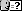Keep the Planet clean! If this comment was disrespectful, please report it:
Reason: